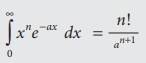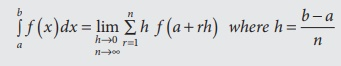# Summary

In this chapter, we have acquired the knowledge of

Summary

In this chapter, we have acquired the knowledge of

·           The relation between the Primitive function and the derived function:

A function F (x) is said to be a primitive function of the derived function f (x) , if d/dx  [ F (x)]= f (x)

·           Integration of a function:

The process of determining an integral of a given function is defined as integration of a function

·           Properties of indefinite integrals:

∫af (x) dx = a ∫ f (x) dx

∫ [f (x) ± g (x) dx = ∫ f (x) dx ± ∫ g (x) dx

·           Standard results of indefinite integrals:·           Definite integral:

Let f ( x) be a continuous function on [a,b] and if F(x) is anti derivative of f (x) , then·           Properties of definite integrals:·           Particular case of Gamma Integral:

If n is a positive integer, then·           Properties of gamma function:

(i) ┌(n) = (n-1) ┌ (n-1) , n > 1

(ii) ┌(n+1) = n┌ (n) , n > 0

(iii) ┌ (n + 1) = n!, n is a positive integer

(iv) ┌ ( 1/2 ) = √π

·           Definite integral as the limit of a sum:

Let f (x) be a continuous real valued function in [a , b], which is divided into n equal parts each of width h , then·           Results:Tags : Integral Calculus - I , 12th Business Maths and Statistics : Chapter 2 : Integral Calculus - I
Study Material, Lecturing Notes, Assignment, Reference, Wiki description explanation, brief detail
12th Business Maths and Statistics : Chapter 2 : Integral Calculus - I : Summary | Integral Calculus - I